# Algebra 1 : How to solve one-step equations

## Example Questions

1 2 46 47 48 49 50 51 52 54 Next →

### Example Question #531 : How To Solve One Step Equations

Solve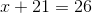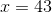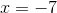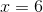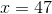Explanation:

Solving for a one-step linear equation is simple. All you're being asked is to solve for x. In other words, how do you get x by itself on one side of the equals sign?

In order to solve for these kind of problems, it's important to keep in mind that what you do to one side must be done to the other.

for, we want to get x by itself. The fastest way would be to move the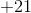from left of the equals sign to the right with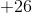.

This can be accomplished by subtractingfrom both sides.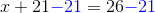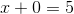### Example Question #532 : How To Solve One Step Equations

Solve for: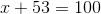47

100

153

57

53

47

Explanation:

Subtract 53 from each side of the equation to getby itself: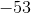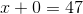1 2 46 47 48 49 50 51 52 54 Next →